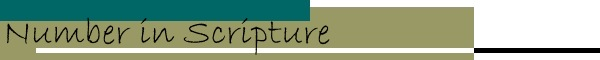Its Supernatural Design and Spiritual Significance
by E.W. Bullinger

### PART II ITS SPIRITUAL SIGNIFICANCE INTRODUCTION

Having thus shown and established the supernatural design in the use of number, both in the works of God and in the Word of God, we now come to the spiritual significance of the numbers themselves.

We propose to take them in order, and to give under each not merely lists of passages or things, but first to define and explain the significance of the number, and then to illustrate its meaning, and show its teaching, as applied to its use, to numbers of things that are mentioned, the numbers of occurrences of words and things, the numbers of words used for a thing, and the numbers formed by the letters of the words themselves. This last is called by the ancients Gematria. This is the use of the letters of the alphabet instead of figures. Arabic numerals being a comparatively modern invention were not, of course, known to, and could not have been used by, the more ancient nations.

The Hebrews and Greeks, therefore, used their alphabets as follows:—

THE HEBREW ALPHABET

consists of 22 (2 x 11) letters, so the 5 finals were added to make up three series of 9, or 27 in all:

 Aleph ) = 1 Yod y = 10 Koph q = 100 Beth b = 2 Kaph k = 20 Resh r = 200 Gimel g = 3 Lamed l = 30 Shin # = 300 Daleth d = 4 Mem m = 40 Tau t = 400 He h = 5 Nun n = 50 Final Kaph K = 500 Vau w = 6 Samech s = 60 Final Mem M = 600 Zayin z = 7 Ayin ( = 70 Final Nun N = 700 Cheth x = 8 Pe p = 80 Final Pe P = 800 Teth + = 9 Tsaddi c = 90 Final Tsaddi C = 900

THE GREEK ALPHABET

The Greek letters were 24, and the required number, 27, was made up by using the final "V" or V (called Stigma) for 6, and adding two arbitrary symbols called respectively Koppa, for 90, and Sampsi, for 900.

 Alpha a =1 Iota i = 10 Rho r = 100 Beta b = 2 Kappa k = 20 Sigma s = 200 Gamma g = 3 Lambda l = 30 Tau t = 300 Delta d = 4 Mu m = 40 Upsilon u = 400 Epsilon e = 5 Nu n = 50 Phi f = 500 Stigma V* = 6 Xi x =60 Chi c = 600 Zeta z = 7 Omicron o = 70 Psi y = 700 Eta h = 8 Pi p = 80 Omega w = 800 Theta q = 9 Koppa = 90 Sampsi = 900

* This letter V (called Stigma) is used for the number 6. Why this letter and number should be thus associated we cannot tell, except that both are intimately connected with the ancient Egyptian "mysteries." The three letters SSS (in Greek SSS) were the symbol of Isis, which is thus connected with 666. Indeed the expression of this number, CxV, consists of the initial and final letters of the word XristoV (Christos), Christ, viz., X and V, with the symbol of the serpent between them, X—xV. number04.htm